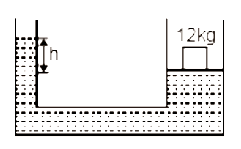#5 | Mercury Barometer
(Physics) > Mechanical Properties of Fluids
Related Practice Questions :

The area of cross-section of the wider tube shown in the figure is  If a mass of 12 kg is placed on the massless piston, the difference is heights h in the level of water in the two tubes is :1.  10 cm

2.  6 cm

3.  15 cm

4.  2 cm

Complete Question Bank + Test Series
Complete Question Bank

Difficulty Level:

If two liquids of same masses but densities ${\mathrm{\rho }}_{1}$ and ${\mathrm{\rho }}_{2}$ respectively are mixed, then density of mixture is given by

(a) $\mathrm{\rho }=\frac{{\mathrm{\rho }}_{1}+{\mathrm{\rho }}_{2}}{2}$                             (b) $\mathrm{\rho }=\frac{{\mathrm{\rho }}_{1}+{\mathrm{\rho }}_{2}}{2{\mathrm{\rho }}_{1}{\mathrm{\rho }}_{2}}$

(c) $\mathrm{\rho }=\frac{2{\mathrm{\rho }}_{1}{\mathrm{\rho }}_{2}}{{\mathrm{\rho }}_{1}+{\mathrm{\rho }}_{2}}$                             (d) $\mathrm{\rho }=\frac{{\mathrm{\rho }}_{1}{\mathrm{\rho }}_{2}}{{\mathrm{\rho }}_{1}+{\mathrm{\rho }}_{2}}$

Complete Question Bank + Test Series
Complete Question Bank

Difficulty Level:

With rise in temperature, density of a given body changes according to one of the following relations

(a)                         (b)

(c)                                 (d)

Complete Question Bank + Test Series
Complete Question Bank

Difficulty Level:

In making an alloy, a substance of specific gravity ${\mathrm{s}}_{1}$ and mass ${\mathrm{m}}_{1}$ is mixed with another substance of specific gravity ${\mathrm{s}}_{2}$ and mass ${\mathrm{m}}_{2}$ ; then the specific gravity of the alloy is

(a) $\left(\frac{{\mathrm{m}}_{1}+{\mathrm{m}}_{2}}{{\mathrm{s}}_{1}+{\mathrm{s}}_{2}}\right)$                               (b) $\left(\frac{{\mathrm{s}}_{1}{\mathrm{s}}_{2}}{{\mathrm{m}}_{1}+{\mathrm{m}}_{2}}\right)$

(c) $\frac{{\mathrm{m}}_{1}+{\mathrm{m}}_{2}}{\left(\frac{{\mathrm{m}}_{1}}{{s}_{1}}+\frac{{m}_{2}}{{\mathrm{s}}_{2}}\right)}$                            (d) $\frac{\left(\frac{{\mathrm{m}}_{1}}{{\mathrm{s}}_{1}}+\frac{{\mathrm{m}}_{2}}{{\mathrm{s}}_{2}}\right)}{{\mathrm{m}}_{1}+{\mathrm{m}}_{2}}$

Complete Question Bank + Test Series
Complete Question Bank

Difficulty Level:

A silver ingot weighing 2.1 kg is held by a string so as to be completely immersed in a liquid of relative density 0.8. The relative density of silver is 10.5. The tension in the string in kg-wt is
(a) 1.6                                             (b) 1.94
(c) 3.1                                             (d) 5.25